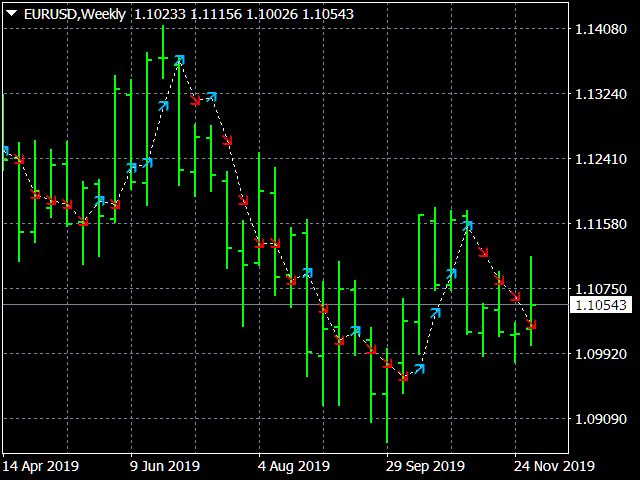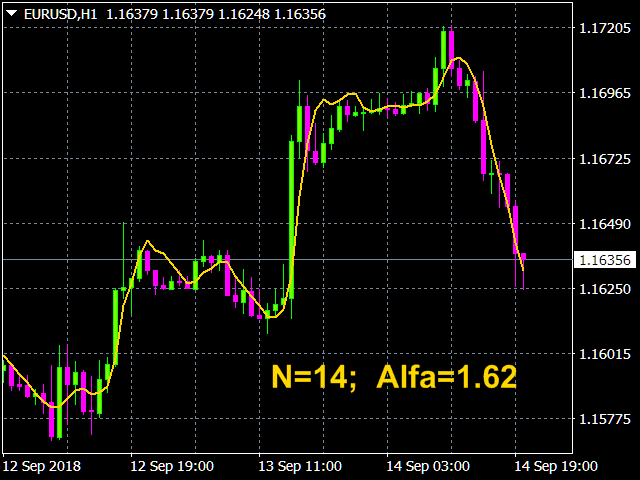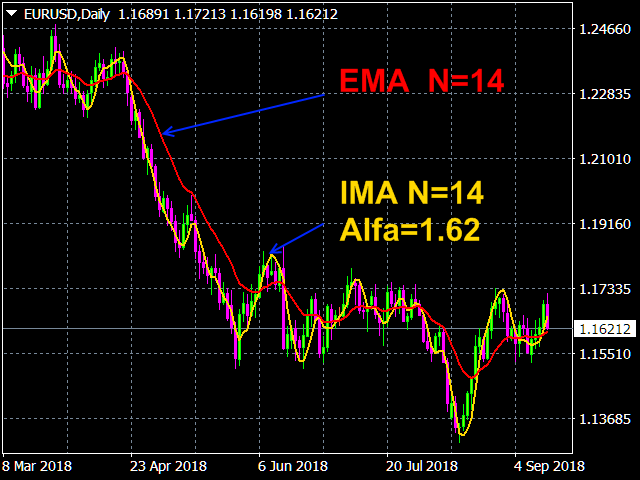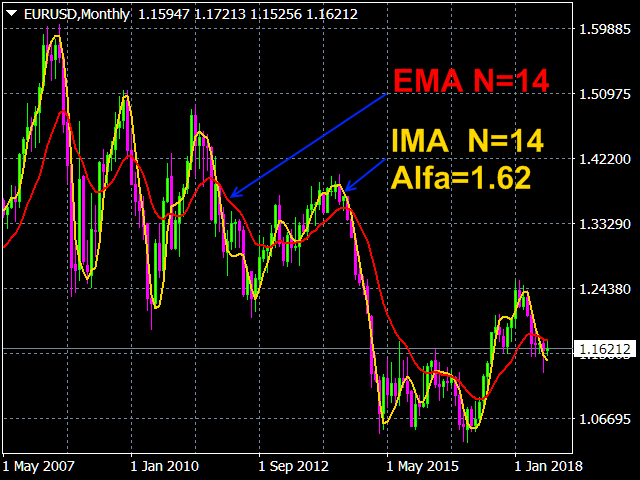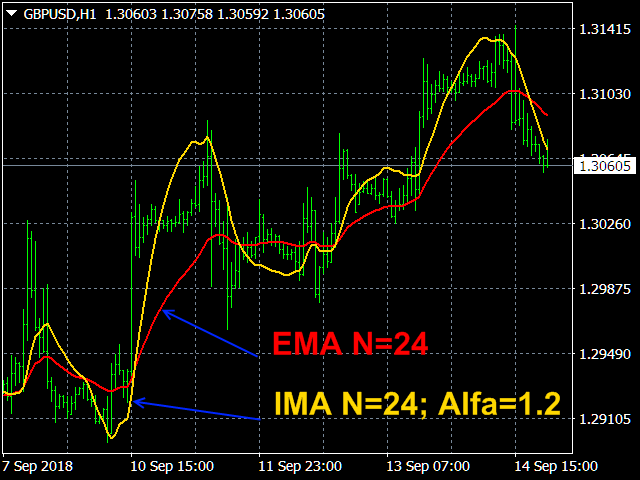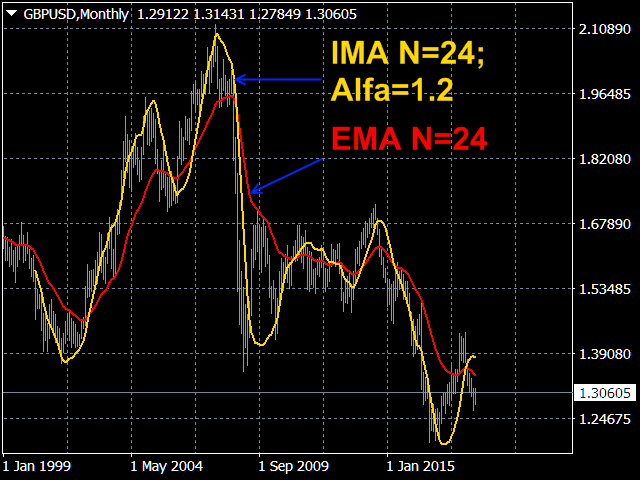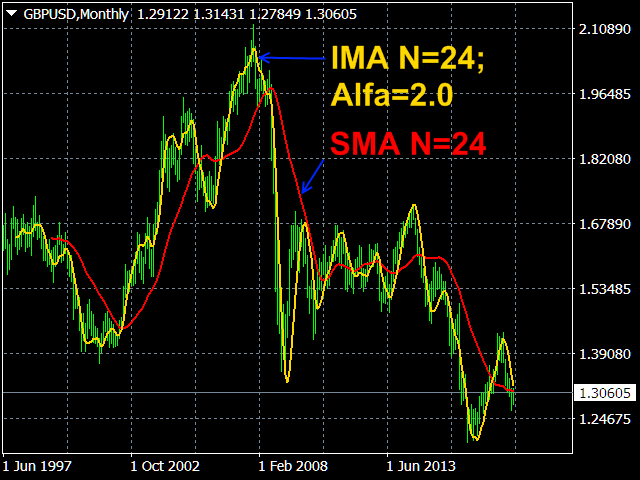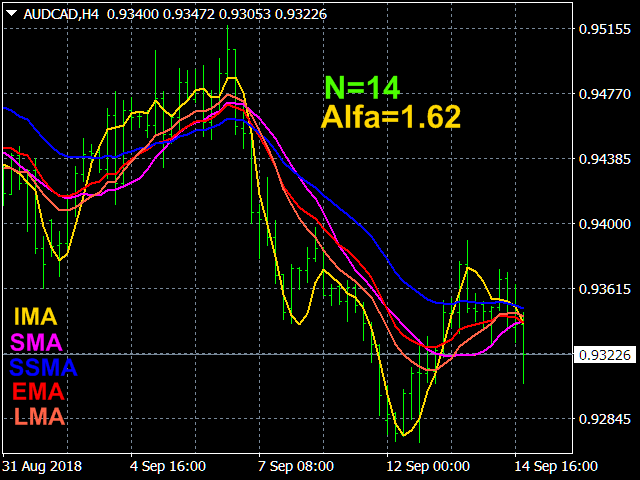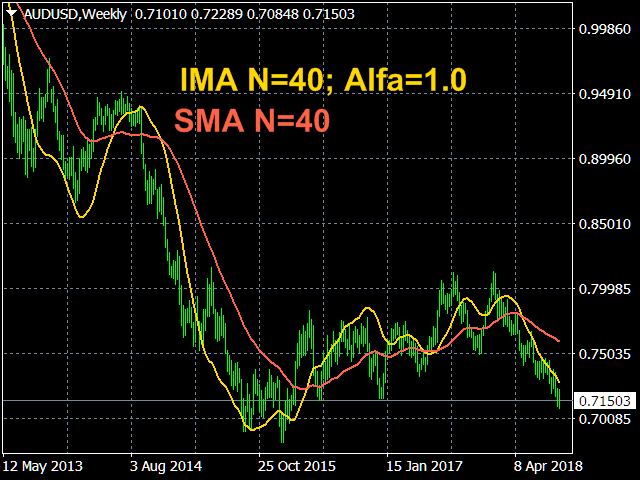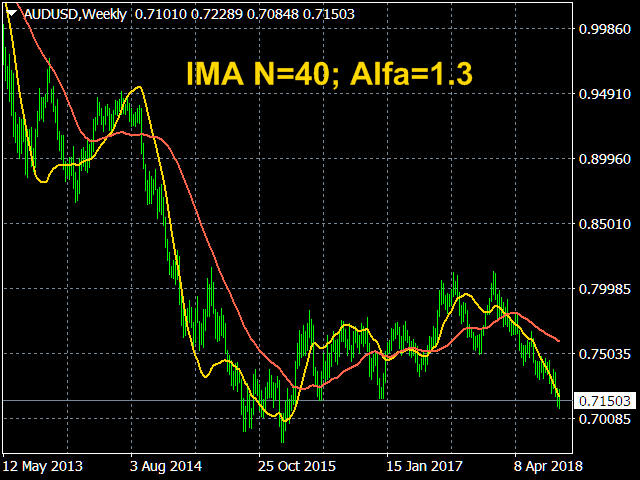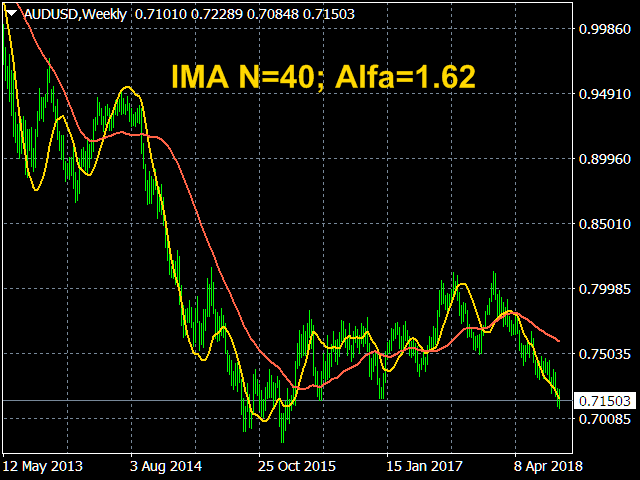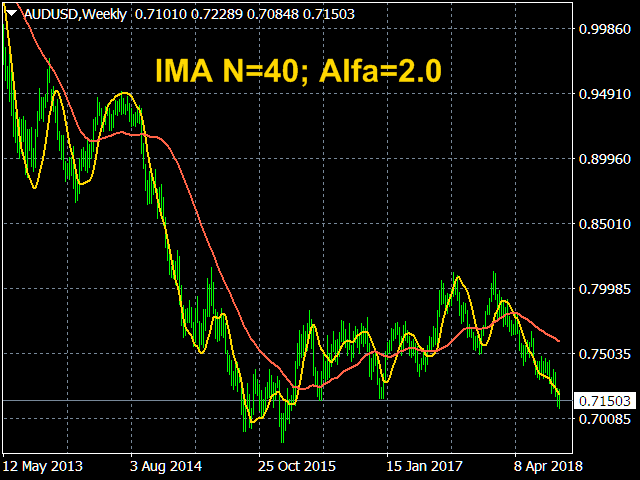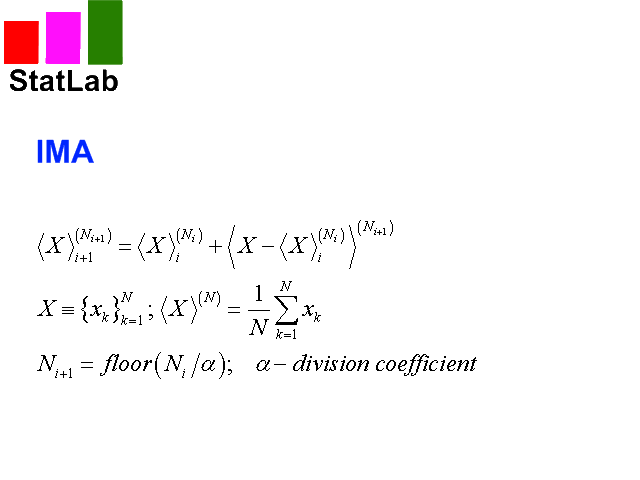Home Stock Market Iterative Moving Average

# Iterative Moving Average

38
0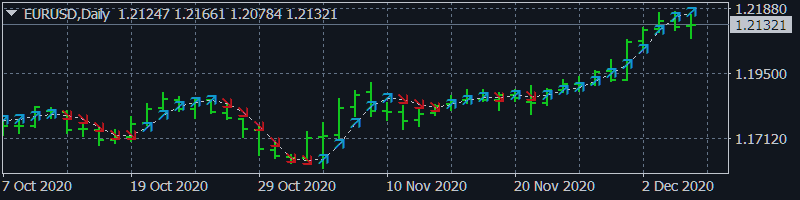Iterative Moving Average – IMA.  IMA is obtained by correcting the usual  MA.  The correction consists in addition  to MA averaged difference between the time series (X) and its MA, i.e.  IMA(X)=MA(X) + MA (Х-MA(X)). Correction is done in several iterations (and, exactly, 2 iterations in this indicator) and with a change in the averaging period.

As a result, the time-series points begin to cluster around (on all sides) of the getting   IMA and with a smaller delay than around the usual MA. Therefore, IMA is a more effective tool for manual and automatic trading than all types of conventional MA  (SMA, EMA, SSMA, LMA).   Formulas for IMA are given in the last screenshot.

### Indicator Settings.

• Price type. Values: Close price, Open price, High price, Low price, Median price ((high + low)/2  – default), Typical price ((high + low + close)/3), Weighted price ((high + low + 2*close)/4).
• The averaging method. Values: Simple, Exponential (default), Smoothed, Linear weighted.
• The main averaging period. Integer. Default is 14.
• Coefficient of division of the averaging period. –  The coefficient determines the period of the next iteration. Values: any positive real number, which is not zero. Default is 1.62 (Golden Ratio).
• Dimensions of markers  – Marker sizes to display forecast points. Values: any positive integer   from 0 to 5 (1 default).

### Screenshots.